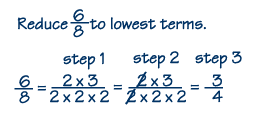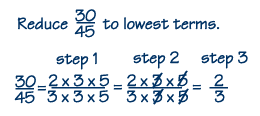Home    |    Teacher    |    Parents    |    Glossary    |    About UsA fraction is reduced to lowest terms, or simplified, when its numerator and denominator have no common factors. It's easier to multiply, divide, add and subtract fractions when they're simplified. To simplify a fraction, we find an equivalent fraction where the numerator and denominator have no common factors. Here are the steps.

1. List the prime factors of the numerator and denominator.
2. Find the factors common to both the numerator and denominator.
3. Divide the numerator and denominator by all common factors (called canceling).

Example 1In step 1, we list the prime factors of the numerator and denominator. In step 2, we divide by, or cancel, the factor of two that is common to both the numerator and denominator. That is, since two divided by two equals one, we say the twos cancel each other. In step 3, we're left with a 3 in the numerator and we multiply the remaining factors in the denominator to get 4. Here's another example.

Example 2In Step 1, we find all the prime factors. In Step 2, we can cancel the common factors 3 and 5.

In Step 3, since the only factors remaining are 2 in the numerator and 3 in the denominator, the final answer is 2/3.

 Homework Help | Pre-Algebra | FractionsEmail this page to a friendSearch·  Definitions·  Reducing fractions·  Adding and subtract-     ing fractions·  Multiplying fractions·  Dividing fractions·  Adding and subtract-     ing mixed numbers·  Multiplying     mixed numbers·  Dividing     mixed numbersFirst Glance In Depth Examples WorkoutReducing fractions